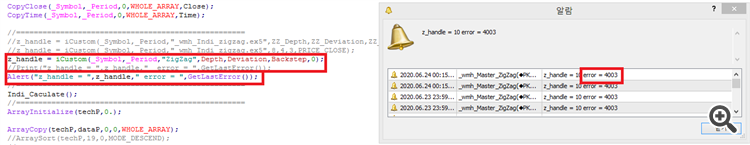# help me error 400317

#property indicator_chart_window

//--- input parameters

input int      Depth          = 12;
input int      Deviation      = 5;

input int      Backstep       = 3;

//-----------

int OnCalculate(const int rates_total,

const int prev_calculated,

const datetime &time[],

const double &open[],

const double &high[],

const double &low[],

const double &close[],

const long &tick_volume[],const long &volume[],

{

z_handle = iCustom(_Symbol,_Period,"ZigZag",Depth,Deviation,Backstep,0);

//Print("z_handle = ",z_handle,"  error = ",GetLastError());

Alert("z_handle = ",z_handle," error = ",GetLastError());40759

What is so hard ? Did you at least check what error 4003 means ?17

Alain Verleyen

Thank You !

I wnat to use indicator of zigzag by icustom.

z_handle = iCustom(_Symbol,_Period,"ZigZag",Depth,Deviation,Backstep,0);

error  = 4603

pls Help me!22306

FireFox: pls Help me!

Why did you repeat what you already said in the original post?

Why didn't you answer his question?

Now answer mine; how many inputs does it have and how many are you passing to iCustom?